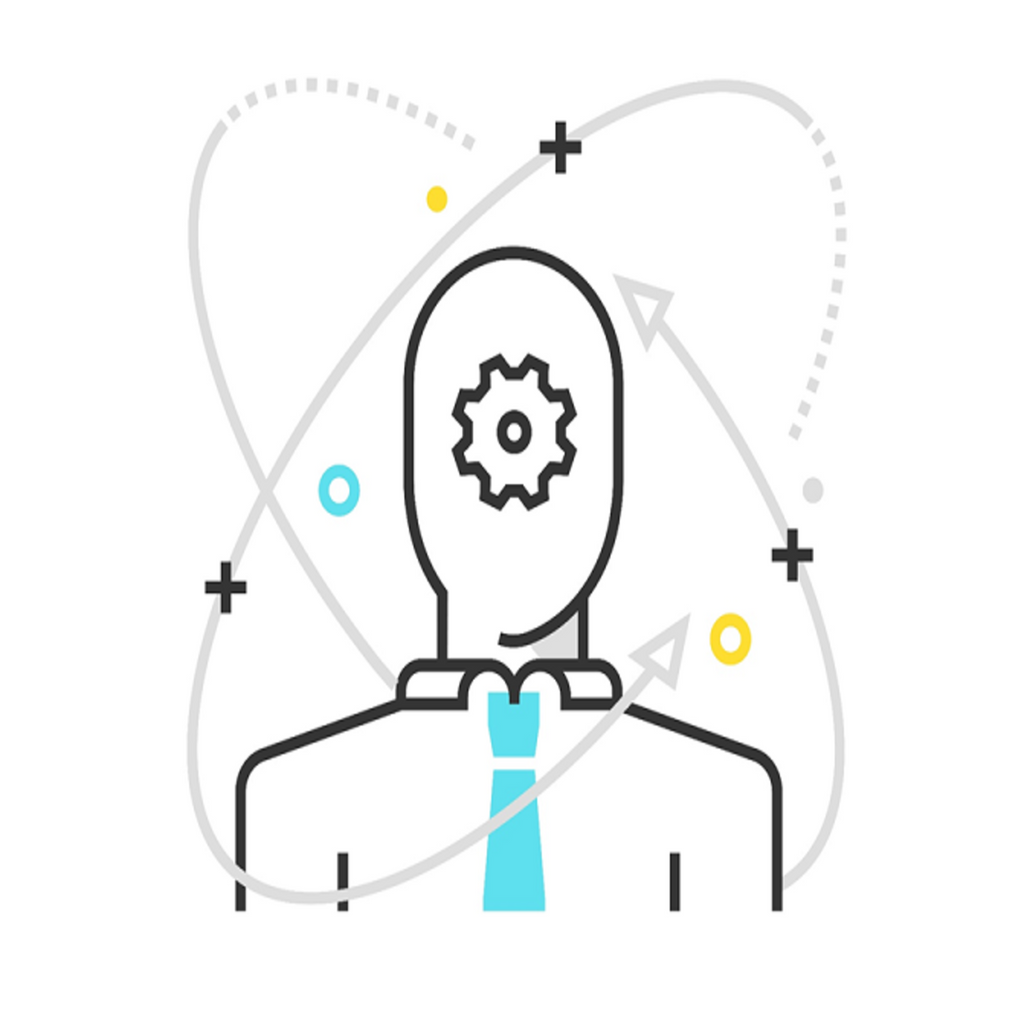Getting Started with Machine Learning with R

\$49.00

description

Machine learning is a subfield of computer science that gives computers the ability to learn without being explicitly programmed. It explores the study and construction of algorithms that can learn from, and make predictions on, data. The R language is widely used among statisticians and data miners to develop statistical software and perform data analysis. Machine Learning is a growing field that focuses on teaching computers to do work that was traditionally reserved for humans; it is a cross-functional domain that uses concepts from statistics, math, software engineering, and more.

In this course, you will start by organizing your data and then predicting it. Then you will work through various examples. The first example will demonstrate (using linear regression) predicting the murder arrest rate based on arrest data for a given State. Here you will explore R Studio and libraries, how to apply linear regression, how to score test sets, and plotting test results on a Cartesian plane. Then the next example will use logistic regression to predict for a classification problem on automobile data: selecting engine cylinders by performance features. This example demonstrates labelling and scaling data, how cross-validation works, and how to apply Logistic regression. Finally, you will move on to the next example—medical data about Diabetes—where you will use the caret package in R to simplify some of these steps.

By the end of this course, you will have mastered preparing data and the tools involved: regression and classification. Additionally, you will have learned to make predictions on new observations.

Style and Approach

In a step-by-step manner, these videos will cover exploratory analysis, creating test/train data sets, algorithms, and then the relevant Machine Learning tools in R. The videos will also make you aware of available ML packages in R. A variety of real-world problem types will be used to illustrate these concepts.

What You Will Learn

In this course you will learn how to:

• Organize and set up your data, and make predictions
• Apply a variety of tools: regression, and classification
• Label and scale data and how cross-validation works
• Make predictions on new observations
• Use the caret package to apply and score a modelGetting Started with Machine Learning with R

Retail value \$49.00